Latest SSC jobs   »   Heights and Distances   »   Heights and Distances

# Heights and Distances Definition, Formula, Trigonometry, Examples

## Heights and Distances

Heights and Distances: Heights and Distances are an important part of trigonometry used in many applications to calculate the height and distance of objects using trigonometric ratios. It has many daily life applications such as calculating the height of building, mountains, towers, or any other object, the depth of the object, the distance between two heavenly bodies, etc. It is also used to find the angle formed between two sides using trigonometric ratios and formulas.

Here we are going to discuss heights and distances including the height and distances formula, trigonometric applications, and problems based on heights and distances. So read the full article to get detailed information about height and distance and their applications.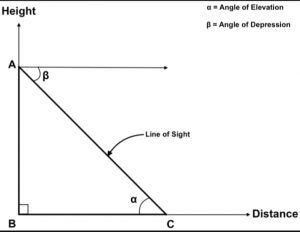## Heights and Distances – Meaning

Height is the measurement of vertical distance from any reference object while distance is the horizontal measurement of any particular point situated horizontally from the object. The height of the building, mountain, tower, flag, etc., and the distance between any two objects can be measured easily using trigonometric ratios and formulas.

## Heights and Distances – Important Terms

You should know some important terms associated with height and distances to understand the concepts of heights and distances. The important terms of heights and distances are as follows:

• Line of Sight – The imaginary horizontal straight line drawn from the observer’s eye to the objects to be viewed. It gives an accurate idea of where the observers view.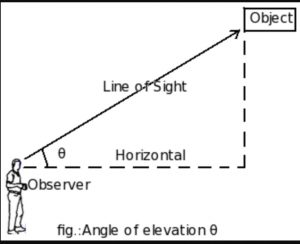• Horizontal line – The line passing parallel to the ground or surface is called a horizontal line. The horizontal line is shown in the above figure.
• The angle of ElevationThe angle formed by the light of sight with the horizontal line when observers view the object situated upward over the horizontal line. It is formed only when the observer views the upward-placed object.• The angle of Depression – The angle of depression is the inclination of light of sight and horizontal line when observers view the downward object. It is formed only when the observer views the object at the ground from any height.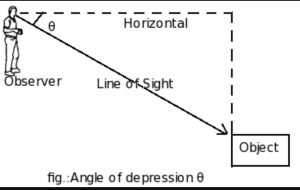## Heights and Distances Formula

The height and distance can be simply calculated using trigonometric ratios. Generally, the Tan trigonometric function is used to get height and distance accurately.

We know that the ratio of the Tan function is tan = Height/Distance

= Perpendicular/Base

You know the values of the tan function at different angles for this. The trigonometric values are mentioned in the table below. You can refer to the table for this.

Note: tan0°=0, tan30°=1/√3, tan45°=1

## Heights and Distances in Trigonometry

Heights and Distances is a very broad application of trigonometry. Using this the distance between two or more objects or places and the height of the object by finding the angle subtended by two sides are found. The height and distance can be calculated using trigonometric functions. Trigonometry and its applications like heights and distances are used by architects, astronauts, surveyors, navigators, etc.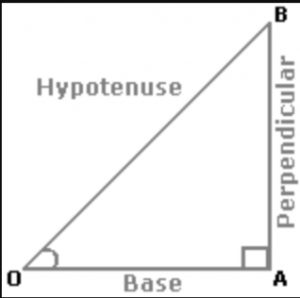### Trigonometric Ratios

The trigonometric Ratios and formulae are mentioned below:

• Sinθ = perpendicular/hypotenuse = AB/OB
• Cosθ = base/hypotenuse = OA/OB
• Tanθ = perpendicular/base = AB/OA
• Cosecθ = 1/Sinθ = OB/AB
• Secθ = 1/Cosθ = OB/OA
• Cotθ = 1/Tanθ = OA/AB

### Trigonometric Identities

1. sin^2θ + cos^2θ= 1

2. 1 + tan^2θ = sec^2θ

3. 1+ cot^2θ =cosec^2θ

### Problems on heights and distances

1. From a point 350m away from the foot of the tower, the topmost point of the tower is observed by an observer making an angle of elevation of 45°. Find the height of the tower in meters?

Solution:   In the right-angled triangle ABC

Tan45°=X/350
X=350m
So the height of the tower is 350m

Que.2 On the ground level the angle of elevation from the top of the tower is 30°. On moving 30m nearer to the tower the angle of elevation is 45° formed. Find the height of the tower?

Solution: Let the height of the tower is h30=h (cot 30°- cot 60°)30=h (√3-1/√3)30√3=h (3-1) h=15√3

Que. 3 Two ships are sailing in the sea on the two sides of a lighthouse. The angle of elevation of the top of the lighthouse is observed from the ships are 30° and 45° respectively. If the lighthouse is 100 m high, the distance between the two ships is:

Solution: Let AB be the lighthouse and C and D be the positions of the ships.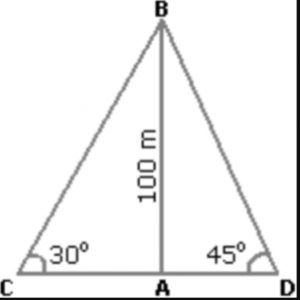Then, AB = 100 m,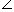ACB = 30° andADB = 45°

AB/AC=tan30°=1/√3

AC = AB√3 = 100√3

CD = (AC + AD) = (100√3 + 100)m

= 100 (√3 + 1)

= 273m

Que.4 The angle of elevation of a ladder leaning against a wall is 60° and the foot of the ladder is 4.6 m away from the wall. The length of the ladder is:

Solution: Let AB be the wall and BC be the ladder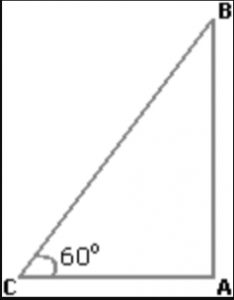Then,ACB = 60° and AC = 4.6 m

AC/BC = cos60° = 1/2

BC = 2×AC

= (2×4.6)m

= 9.2m

Que.5 The angles of depression of two ships from the top of a lighthouse are 45° and 30° towards east. if the ships are 200 m apart, find the height of the light house.

Solution: Let us draw a figure below as per given question

Let AB = h meter be the height of the tower and two ships are situated at D and C respectively; such that, CD = 200 m; ∠ADC = 30° ∠ACB = 45° and BC = x meter (say)
Now from right triangle ABC,
tan 45° = h/x ⇒ 1 = h/x
∴ x = h
Again from right triangle ABD,
tan 30° = h/( 200 + x )
⇒ 1/√3 = h/( 200 + x )
Since x = h , we will get.
⇒ 1/√3 = h/(200 + h )
⇒ (200 + h ) = h X √3
⇒ h X √3 – h = 200
⇒ h x 1.732 – h = 200
⇒ h(1.732 – 1) = 200
∴ h = 200/0.732 = 273.2 m = 273 m

Que.6 At a point on a level plane a tower subtends an angle Θ and a flag-staff a ft. in length at the top of the tower subtends an angle Θ . The height of the tower is :

Solution: Let us draw a figure as per given question.
Let OP be the tower of height h (say) and PQ the flag-staff of height a, such that OAP = Θ and PAQ = Φ
In ΔOAP

TanΘ = P/B = Perpendicular distance / Base distance
⇒ TanΘ = OP/OA
⇒ OA = OP CotΘ
Put the value of OP in above equation, we will get
⇒ OA = hCotΘ ……………. (1)
Now from triangle ΔOAQ,
⇒ Tan(Θ + Φ) = OQ/OA
⇒ OA = OQ Cot(Θ + Φ)
⇒ OA = (h + a) Cot(Θ + Φ) ………….(2)
from equation (1) and (2), We will get
∴ hCotΘ = (h + a) Cot(Θ + Φ)
⇒ CotΘ / Cot(Θ + Φ) = (h + a) / h
⇒ CotΘ / Cot(Θ + Φ) = h / h + a / h
⇒ CotΘ / Cot(Θ + Φ) = 1 + a / h
⇒ (CotΘ / Cot(Θ + Φ)) – 1 = a / h
⇒ (CotΘ – Cot(Θ + Φ) ) / Cot(Θ + Φ) = a / h
⇒ h = aCot (Θ + Φ) / CotΘ – Cot(Θ + Φ)
After converting Cot into Sin and Cos, We will get
⇒ h = aSinΘCos(Θ + Φ)/SinΦ

Que.7 An observer 1.6 m tall is 20√3 away from a tower. The angle of elevation from his eye to the top of the tower is 30°. Find the height of tower?

Solution:

Let observer be AB and tower be CD

BE is perpendicular to CD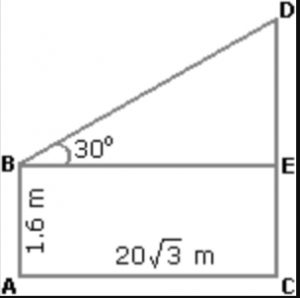AB = CE = 1.6m

AC = BE = 20√3m

DE/BE = tan30° = 1/√3

then DE = 20√3/√3 m

= 20m

Since CD = CE + DE = 1.6 + 20

= 21.6m

Que.8 From a point P on a level ground, the angle of elevation of the top tower is 30°. If the tower is 100 m high, find the distance of point P from the foot of tower?

Solution: Suppose AB be a tower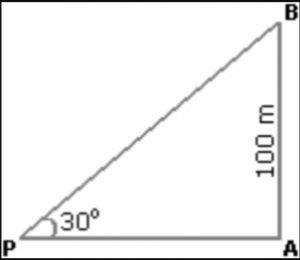Angle APB = tan30° = 1/√3

AP = (AB×√3)m

= 100√3m

= 100×1.73

= 173m

Que.9 The angle of elevation of the sun, when the length of the shadow of a tree 3 times the height of the tower, is?

Solution:

Suppose AB be a tree and AC be its shadow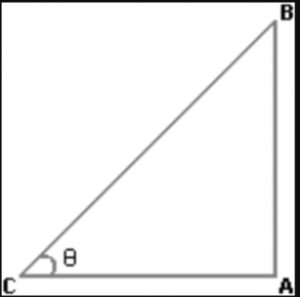Let angle ACB = theta

AC/AB = √3

Cot = √3

= 30°

Que.10 A man is standing on the deck of a ship, which is 10m above water level. He observes the angle of elevation of the top of a light house as 600 and the angle of depression of the base of lighthouse as 300. Find the height of the light house.

Solution:

Let AB is the light house and the man is standing at C so, ∠BCD = 600 and ∠ACD = 300.

Let BD = h

In ΔADC, tan 300 = 10/CD

⇒ 1/√3 = 10/CD ⇒ CD = 10√3m

In ΔBDC, tan 600 = h/CD

⇒√3 = h/10√3

⇒ h = 30m

So the height of the light house is AB = AD + BD = 10 + 30 = 40m

### Heights and Distances- FAQs

Que.1 Define height and distance.

Ans – Height is defined as the vertical distance between two objects while distance is the measurement of any point or object in a particular direction.

Que.2 What is angle of elevation?

Ans – It is the angle formed between line of sight and ground level when observer views the object situated at ground level from any particular height.

Que.3 Define the term angle of depression?

Ans – The Angle of depression is the inclination of line of sight from a horizontal line when the observer views the object placed downward.

Que.4 What are the application of height and distances?

Ans – Heights and distances are a part of trigonometry and have many applications in daily life practices. It is used to calculate the height of towers, buildings, mountains, etc., and the distance between any two objects like celestial bodies or others. It is mostly used by astronauts, surveyors, architects, and navigators.

#### Congratulations!General Awareness & Science Capsule PDF•What is Frequency Polygon? Statistics, G...
•SSC CGL Quantitative Aptitude : 19th Feb...